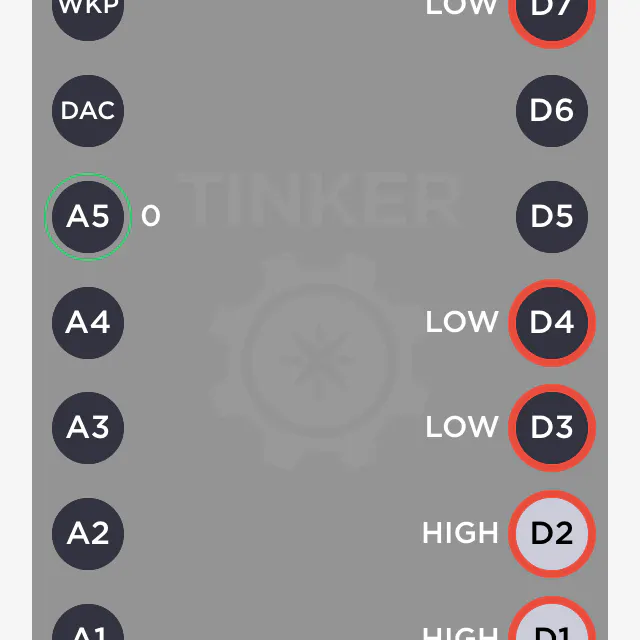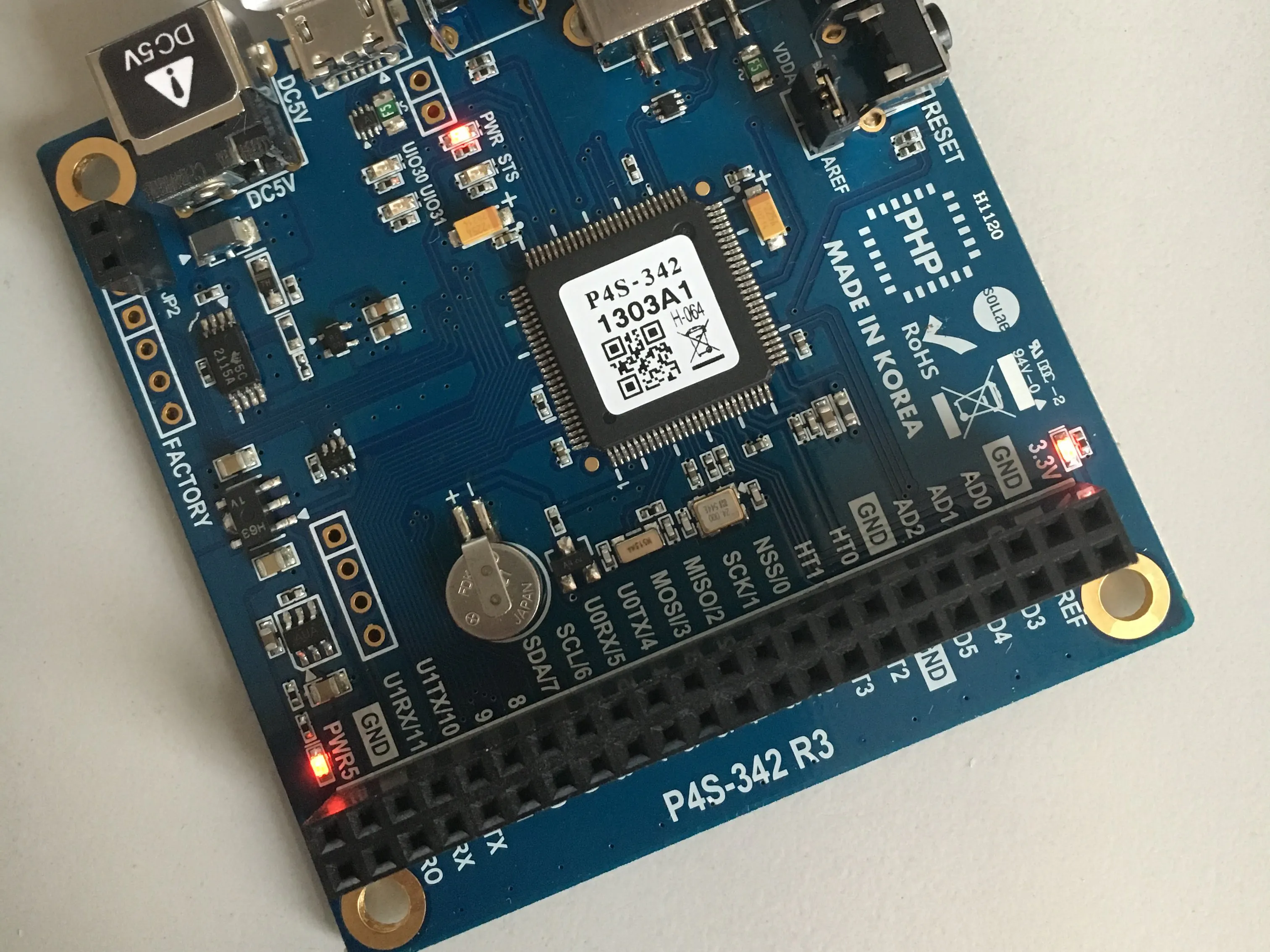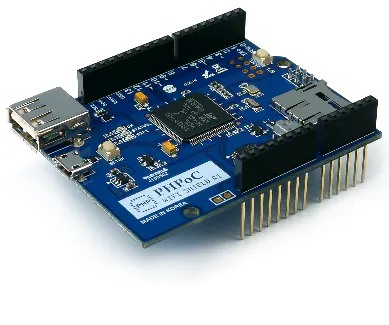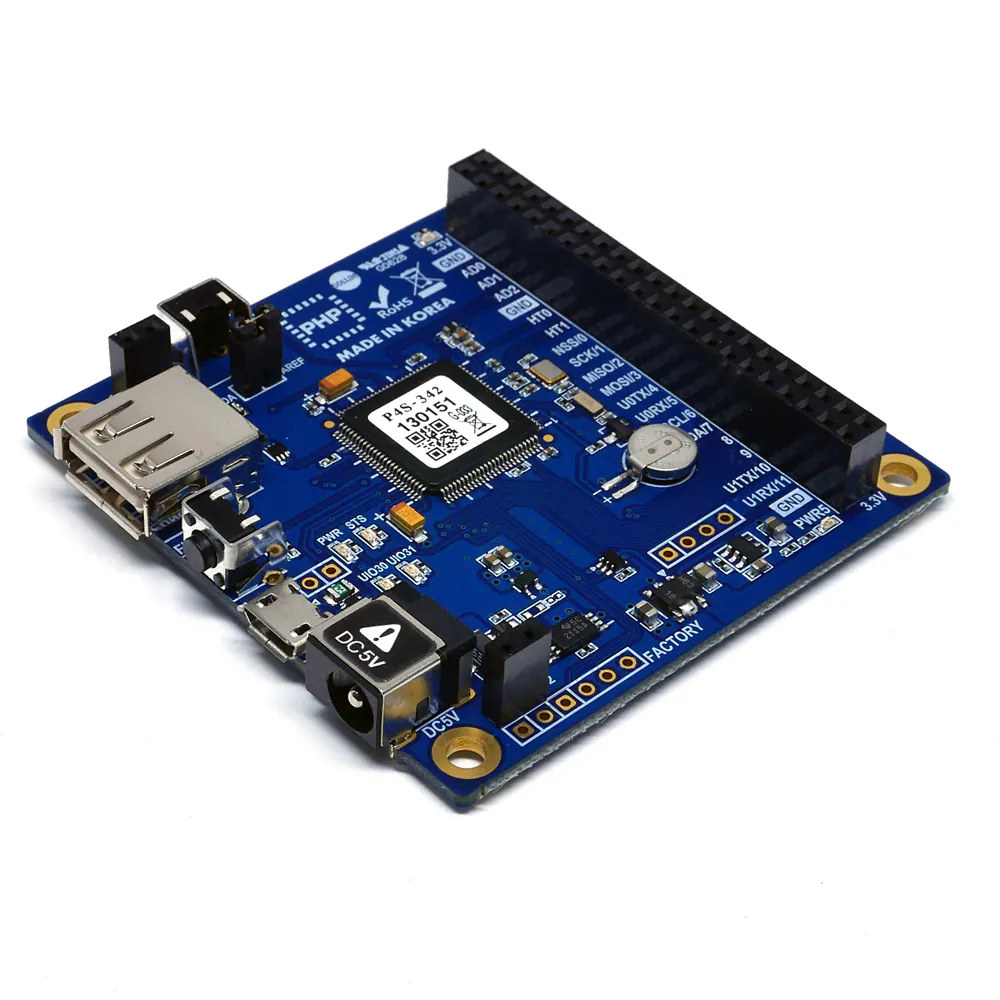# PHPoC: Preparation & Introduction

If you have PHP knowledge, then this introduction to PHPoC (PHP on Chip) is for you.

BeginnerProtip2 hours1,636## Things used in this project

### Hardware componentsPHPoC WiFi Shield for Arduino a Shield for Arduino Uno/Mega
×1PHPoC Blue
×1

### Software apps and online services## Code

### Arduino Sketch

C/C++
```/* arduino web server - remote control (slide switch) */
// original phpoc-example with 2 changes:
// definition for a LED PIN at 9 and
// if-clause to check the slider
// Ingo Lohs, 24.10.2018

#include "SPI.h"
#include "Phpoc.h"

PhpocServer server(80);

char slideName;
int slideValue;

void setup() {
Serial.begin(9600);
while(!Serial);

Phpoc.begin(PF_LOG_SPI | PF_LOG_NET);
//Phpoc.begin();

server.beginWebSocket("remote_slide");

Serial.println(Phpoc.localIP());

analogWrite (9, 127); // Port an the Shield and default Value for Brightness in the Range 0-255 from dark to max lightning
}

void loop() {
// wait for a new client:
PhpocClient client = server.available();

if (client) {

if(slideStr)
{
slideName = slideStr.charAt(0);
slideValue = slideStr.substring(1).toInt();

// if-clause for changed values from php-form
if(slideName == 'A')
{
analogWrite (9, slideValue);
}

Serial.print(slideName);
Serial.print('/');
Serial.println(slideValue);
}
}
}
```

### PHPoC Shield for Arduino: remote_slide_led.php

PHP
```<?php
include_once "config.php";
include_once "/lib/sc_envu.php";
if(!(\$wrs_width = envu_find(\$envu, "wrs_width")))
\$wrs_width = "150";
if(!(\$wrs_length = envu_find(\$envu, "wrs_length")))
\$wrs_length = "300";
if(!(\$wrs_value_min = envu_find(\$envu, "wrs_value_min")))
\$wrs_value_min = "0";
if(!(\$wrs_value_max = envu_find(\$envu, "wrs_value_max")))
\$wrs_value_max = "255";
?>
<!DOCTYPE html>
<html>
<title>PHPoC Shield - Web Remote Control for Arduino</title>
<meta name="viewport" content="width=device-width, initial-scale=0.7, maximum-scale=0.7">
<style>
body { text-align: center; font-size: 15pt; }
h1 { font-weight: bold; font-size: 25pt; }
h2 { font-weight: bold; font-size: 15pt; }
button { font-weight: bold; font-size: 15pt; }
</style>
<script>
var SLIDE_WIDTH = <?echo(int)\$wrs_width/2?>;
var SLIDE_LENGTH = <?echo(int)\$wrs_length?>;
var VALUE_MIN = <?echo(int)\$wrs_value_min?>;
var VALUE_MAX = <?echo(int)\$wrs_value_max?>;
var BUTTON_WIDTH = parseInt(SLIDE_WIDTH * 0.8);
var BUTTON_HEIGHT = parseInt(BUTTON_WIDTH / 2);
var SLIDE_HEIGHT = parseInt(SLIDE_LENGTH + BUTTON_HEIGHT * 1.1);
var slide_info = [ null, null ];
var ws;
function init()
{
var remote = document.getElementById("remote");

remote.width = SLIDE_WIDTH;
remote.height = SLIDE_HEIGHT;
remote.style = "border:1px solid black";

slide_info = {x:0, y:0, offset:0, state:false, identifier:null, ws_value:0};

slide_info.x = parseInt(SLIDE_WIDTH / 2);
slide_info.y = parseInt(SLIDE_HEIGHT / 2);

update_slide(0, SLIDE_HEIGHT / 2);

}
function connect_onclick()
{
if(ws == null)
{
var debug = document.getElementById("debug");

if((navigator.platform.indexOf("Win") != -1) && (ws_host_addr.charAt(0) == "["))
{
// network resource identifier to UNC path name conversion
}

//debug.innerHTML = "<br>" + navigator.platform + " " + ws_host_addr;
ws = new WebSocket("ws://" + ws_host_addr + "/remote_slide", "text.phpoc");

document.getElementById("ws_state").innerHTML = "CONNECTING";

ws.onopen = ws_onopen;
ws.onclose = ws_onclose;
ws.onmessage = ws_onmessage;
}
else
ws.close();
}
function ws_onopen()
{
document.getElementById("ws_state").innerHTML = "<font color='blue'>CONNECTED</font>";
document.getElementById("bt_connect").innerHTML = "Disconnect";

// draw active slide button
for(slide_id = 0; slide_id < 1; slide_id++)
{
var slide = slide_info[slide_id];

update_slide(slide_id, slide.y - slide.offset);
}
}
function ws_onclose()
{
document.getElementById("ws_state").innerHTML = "<font color='gray'>CLOSED</font>";
document.getElementById("bt_connect").innerHTML = "Connect";

ws.onopen = null;
ws.onclose = null;
ws.onmessage = null;
ws = null;

// draw inactive slide button
for(slide_id = 0; slide_id < 1; slide_id++)
{
var slide = slide_info[slide_id];

update_slide(slide_id, slide.y - slide.offset);
slide.ws_value = 0;
}
}
function ws_onmessage(e_msg)
{
e_msg = e_msg || window.event; // MessageEvent

}
function update_slide(slide_id, y)
{
var debug = document.getElementById("debug");
var remote = document.getElementById("remote");
var ctx = remote.getContext("2d");
var slide = slide_info[slide_id];
var slide_top, slide_ratio, slide_value;

slide_top = (SLIDE_HEIGHT - SLIDE_LENGTH) / 2;

slide.y = y + slide.offset;

if(slide.y < slide_top)
slide.y = slide_top;

if(slide.y > (slide_top + SLIDE_LENGTH))
slide.y = slide_top + SLIDE_LENGTH;

ctx.clearRect(SLIDE_WIDTH * slide_id, 0, SLIDE_WIDTH, SLIDE_HEIGHT);

ctx.fillStyle = "silver";
ctx.beginPath();
ctx.rect(slide.x - 5, slide_top, 10, SLIDE_LENGTH);
ctx.fill();

{
ctx.strokeStyle = "blue";
if(slide.state)
ctx.fillStyle = "blue";
else
ctx.fillStyle = "skyblue";
}
else
{
ctx.strokeStyle = "gray";
if(slide.state)
ctx.fillStyle = "gray";
else
ctx.fillStyle = "silver";
}

ctx.beginPath();
ctx.rect(slide.x - BUTTON_WIDTH / 2, slide.y - BUTTON_HEIGHT / 2, BUTTON_WIDTH, BUTTON_HEIGHT);
ctx.fill();
ctx.stroke();

ctx.font = "30px Arial";
ctx.textBaseline = "top";
ctx.fillStyle = "white";

slide_ratio = (SLIDE_LENGTH - (slide.y - slide_top)) / SLIDE_LENGTH;       // 0 ~ 1
slide_value = parseInt(slide_ratio * (VALUE_MAX - VALUE_MIN) + VALUE_MIN); // VALUE_MIN ~ VALUE_MAX

if(slide_id == 0)
{
ctx.textAlign = "right";
ctx.fillText(slide_value.toString(), slide.x + BUTTON_WIDTH / 2 - 5, slide.y - BUTTON_HEIGHT / 2);
}
else
{
ctx.textAlign = "left";
ctx.fillText(slide_value.toString(), slide.x - BUTTON_WIDTH / 2 + 5, slide.y - BUTTON_HEIGHT / 2);
}

{
//debug.innerHTML = slide.ws_value + "/" + slide_value;

if(slide.ws_value != slide_value)
{
if(slide_id == 0)
ws.send("A" + slide_value.toString() + "\r\n");
else
ws.send("B" + slide_value.toString() + "\r\n");

slide.ws_value = slide_value;
}
}
}
function find_slide_id(x, y)
{
var button_left, button_right, button_top, button_bottom;
var slide_id, slide;

if(x < SLIDE_WIDTH)
slide_id = 0;
else
slide_id = 1;

slide = slide_info[slide_id];

button_left = slide.x - BUTTON_WIDTH / 2;
button_right = slide.x + BUTTON_WIDTH / 2;
button_top = slide.y - BUTTON_HEIGHT / 2;
button_bottom = slide.y + BUTTON_HEIGHT / 2;

if((x > button_left) && (x < button_right) && (y > button_top) && (y < button_bottom))
return slide_id;
else
return 2;
}
function mouse_down(event)
{
var debug = document.getElementById("debug");
var x, y, slide_id;

//debug.innerHTML = "";

if(event.changedTouches)
{
for(var id = 0; id < event.changedTouches.length; id++)
{
var touch = event.changedTouches[id];

x = touch.pageX - touch.target.offsetLeft;
y = touch.pageY - touch.target.offsetTop;

slide_id = find_slide_id(x, y);

//debug.innerHTML += slide_id + "/" + x + "/" + y + " ";
//debug.innerHTML += slide_id + "/" + touch.identifier + " ";

if(slide_id < 2)
{
var slide = slide_info[slide_id];

if(!slide.state)
{
slide.offset = slide.y - y;
slide.identifier = touch.identifier;
slide.state = true;

update_slide(slide_id, y);
}
}
}
}
else
{
x = event.offsetX;
y = event.offsetY;

slide_id = find_slide_id(x, y);

if(slide_id < 2)
{
var slide = slide_info[slide_id];

slide.offset = slide.y - y;
slide.state = true;

update_slide(slide_id, y);
}
}

event.preventDefault();
}
function mouse_up(event)
{
var debug = document.getElementById("debug");
var slide_id;

//debug.innerHTML = "";

if(event.changedTouches)
{
for(var id = 0; id < event.changedTouches.length; id++)
{
var touch = event.changedTouches[id];

if(touch.identifier == slide_info.identifier)
slide_id = 0;
else
if(touch.identifier == slide_info.identifier)
slide_id = 1;
else
slide_id = 2;

if(slide_id < 2)
{
var slide = slide_info[slide_id];

slide.state = false;
slide.identifier = null;

if(document.getElementById("bt_center").checked == true)
{
slide.offset = 0;
update_slide(slide_id, SLIDE_HEIGHT / 2);
}
else
update_slide(slide_id, slide.y - slide.offset);
}
}
}
else
{
if(slide_info.state)
slide_id = 0;
else
if(slide_info.state)
slide_id = 1;
else
slide_id = 2;

if(slide_id < 2)
{
var slide = slide_info[slide_id];

slide.state = false;

if(document.getElementById("bt_center").checked == true)
{
slide.offset = 0;
update_slide(slide_id, SLIDE_HEIGHT / 2);
}
else
update_slide(slide_id, slide.y - slide.offset);
}
}

event.preventDefault();
}
function mouse_move(event)
{
var debug = document.getElementById("debug");
var x, y, slide_id, offset;

//debug.innerHTML = "";

if(event.changedTouches)
{
for(var id = 0; id < event.changedTouches.length; id++)
{
var touch = event.changedTouches[id];

if(touch.identifier == slide_info.identifier)
slide_id = 0;
else
if(touch.identifier == slide_info.identifier)
slide_id = 1;
else
slide_id = 2;

if(slide_id < 2)
{
x = touch.pageX - touch.target.offsetLeft;
y = touch.pageY - touch.target.offsetTop;

update_slide(slide_id, y);
}
}
}
else
{
if(slide_info.state)
slide_id = 0;
else
if(slide_info.state)
slide_id = 1;
else
slide_id = 2;

if(slide_id < 2)
{
x = event.offsetX;
y = event.offsetY;

update_slide(slide_id, y);
}
}

event.preventDefault();
}
function bt_center_change()
{
if(document.getElementById("bt_center").checked == true)
{
for(slide_id = 0; slide_id < 1; slide_id++)
{
var slide = slide_info[slide_id];

slide.offset = 0;
update_slide(slide_id, SLIDE_HEIGHT / 2);
}
}
}
</script>

<body>

<p>
<h1>Web Remote Control / Slide</h1>
</p>

<canvas id="remote"></canvas>

<h2>WebSocket <font id="ws_state" color="gray">CLOSED</font></h2>
<button id="bt_connect" type="button" onclick="connect_onclick();">Connect</button>
<span id="debug"></span>

</body>
</html>
```

## Credits

### Ingo Lohs

182 projects • 179 followers
I am well over 40 years and come from the middle of Germany.